# Create formula with structured references in WPS Office Excel

February 21, 2022
563 Views 0

## · Explicit reference & Structured reference in WPS Spreadsheet

Before learning how to use structured reference, it’s best to know what it is and why it is used first. Here we introduce another concept Explicit reference for comparison.

### 1. What is Explicit Reference

Explicit Reference is mostly used in our daily work. When you input the specific cell range like C2:E9 in a formula, you are using Explicit Reference.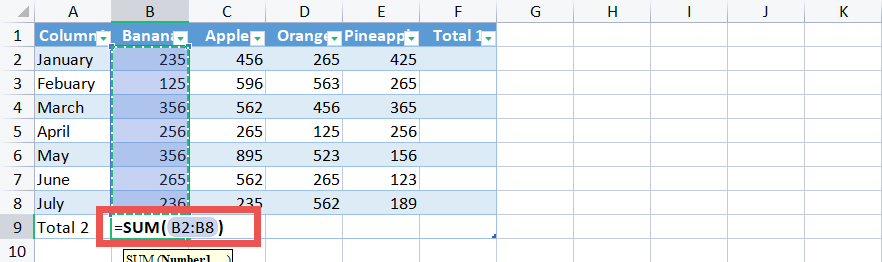The specific cell range you reference can be known easily from the formula, but you may not learn what the formula is calculating.

Besides, if you changed the table, for example, inserting a column, you have to revise your formula.

### 2. What is Structured reference

Explicit Reference refers the combination of the table and column names. When you input the table and column names instead of the specific cell range in a formula, you are using Structured Reference.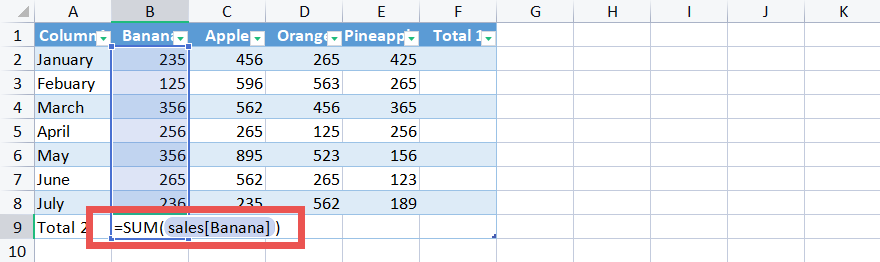## · Steps to use structured references in WPS Spreadsheet

### Step 1: Change the table name for structured references

1. Insert a table in WPS Spreadsheet: Select the cell range of your data, click the Insert tab > Table > check My table has headers > OK.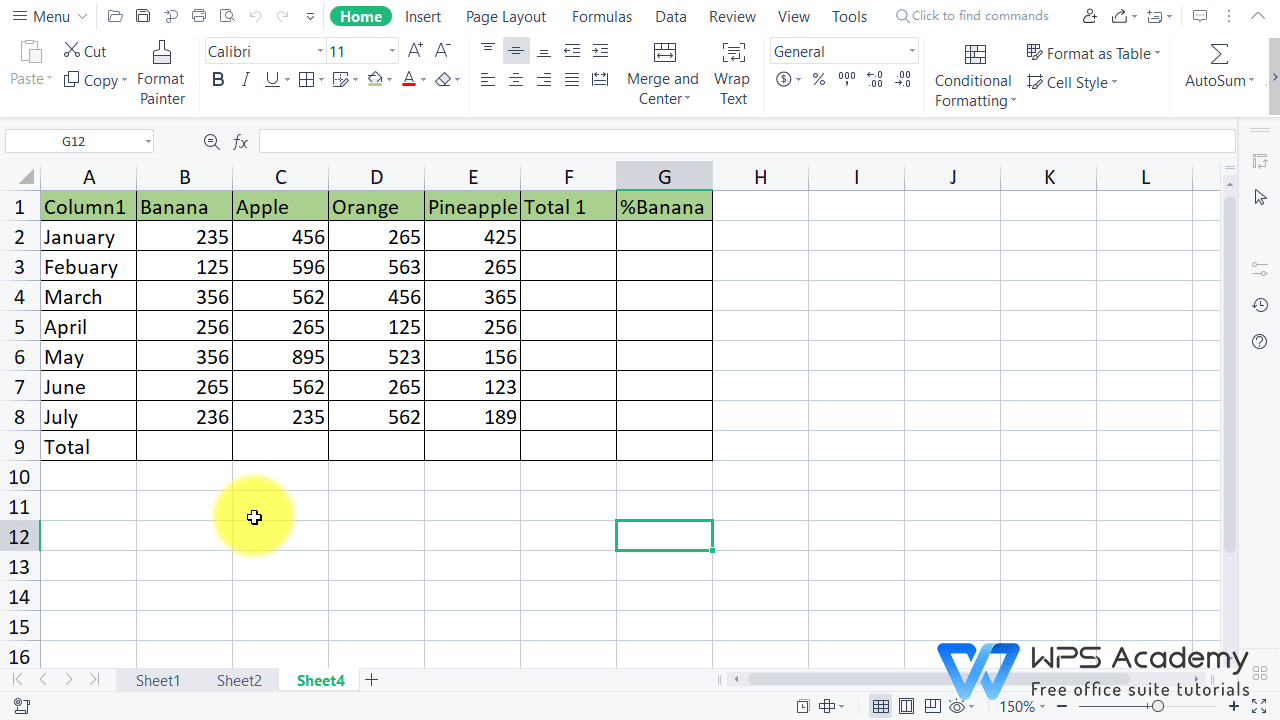2. Click any cell of the table to enter the Table Tools tab, input the Table Name in the upper left corner of the ribbon and press Enter to confirm it.

(Here we input sales for demonstration. You can also use the default name.)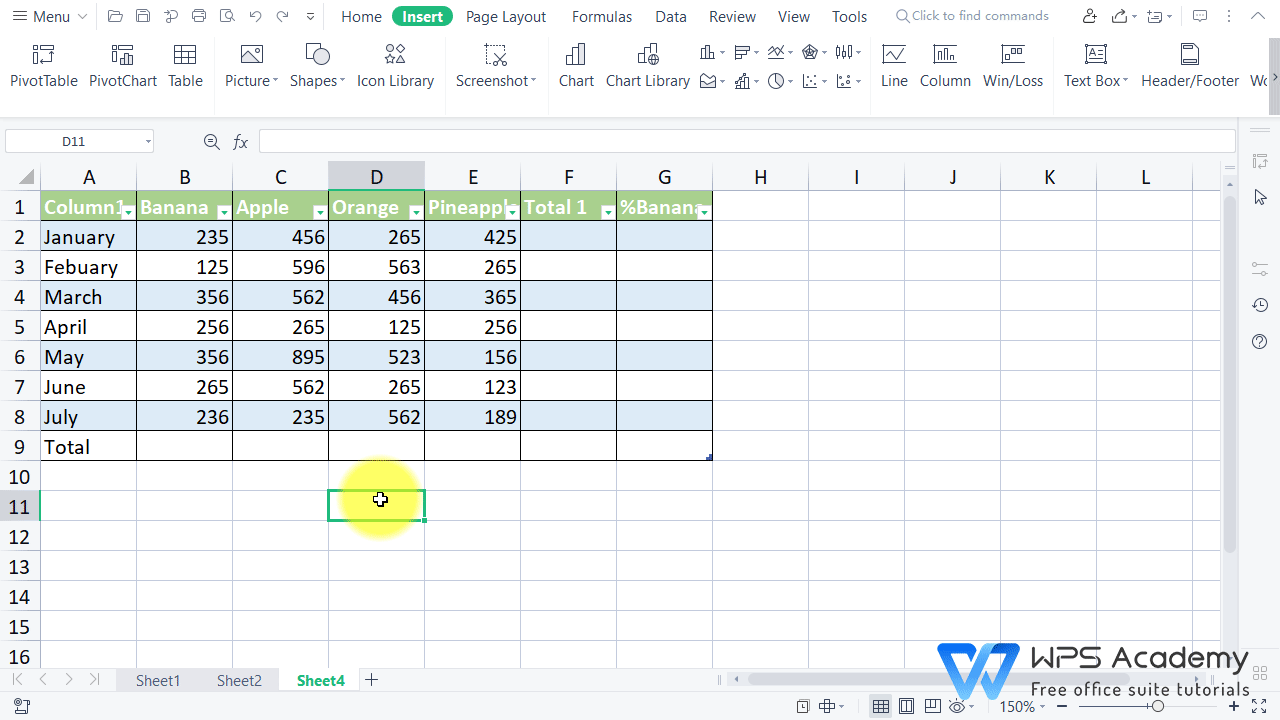### Step 2: Know about the structured reference syntax rules

Here we take two formulas as examples to learn about the syntax rules.

=SUM(Sales[Banana])

Table name: “Sales” is a custom table name. It references the table data, without any header or total rows.

Column specifier: [Banana] and [Apple] are column specifiers that use the names of the columns they represent. They reference the column data, without any column header or total row. Remember to enclose specifiers in brackets.

### Step 3: Apply structured references to a spreadsheet  example

1. Suppose we want to calculate the total sales of Banana. Input: =SUM(Sales[Banana]).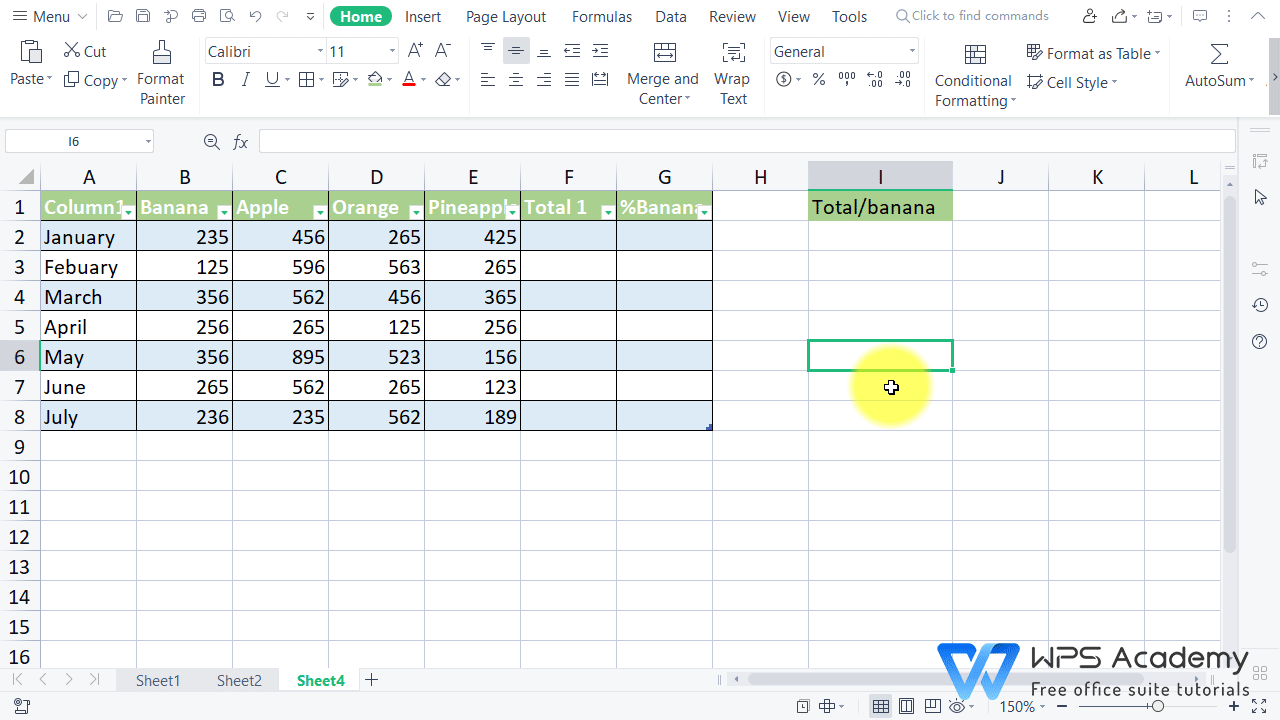2. Suppose we want to calculate the total sales of January. Input: =SUM(Sales[@Banana],[@Apple],[@Orange],[@Pineapple]).

Tips: the @ symbol refers to this row.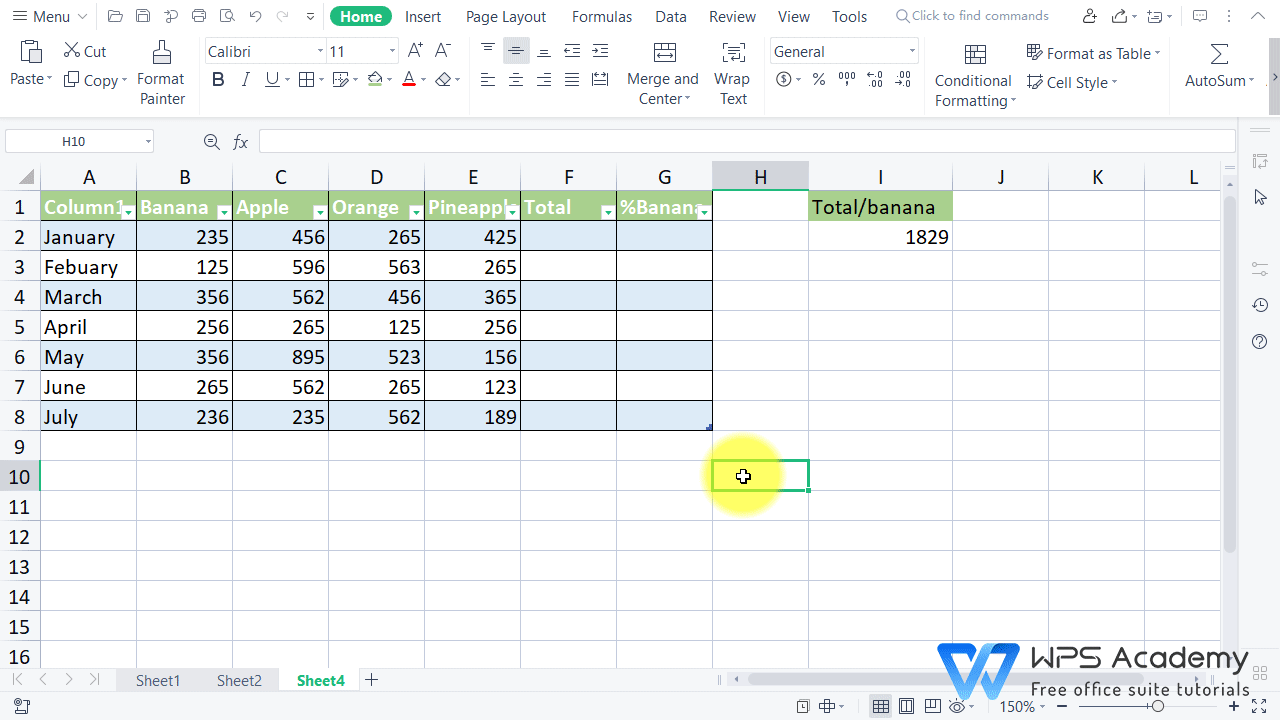3. Suppose we want to calculate the sales proportion of Banana in each month. Input: =[@Banana]/[@Total]*100&%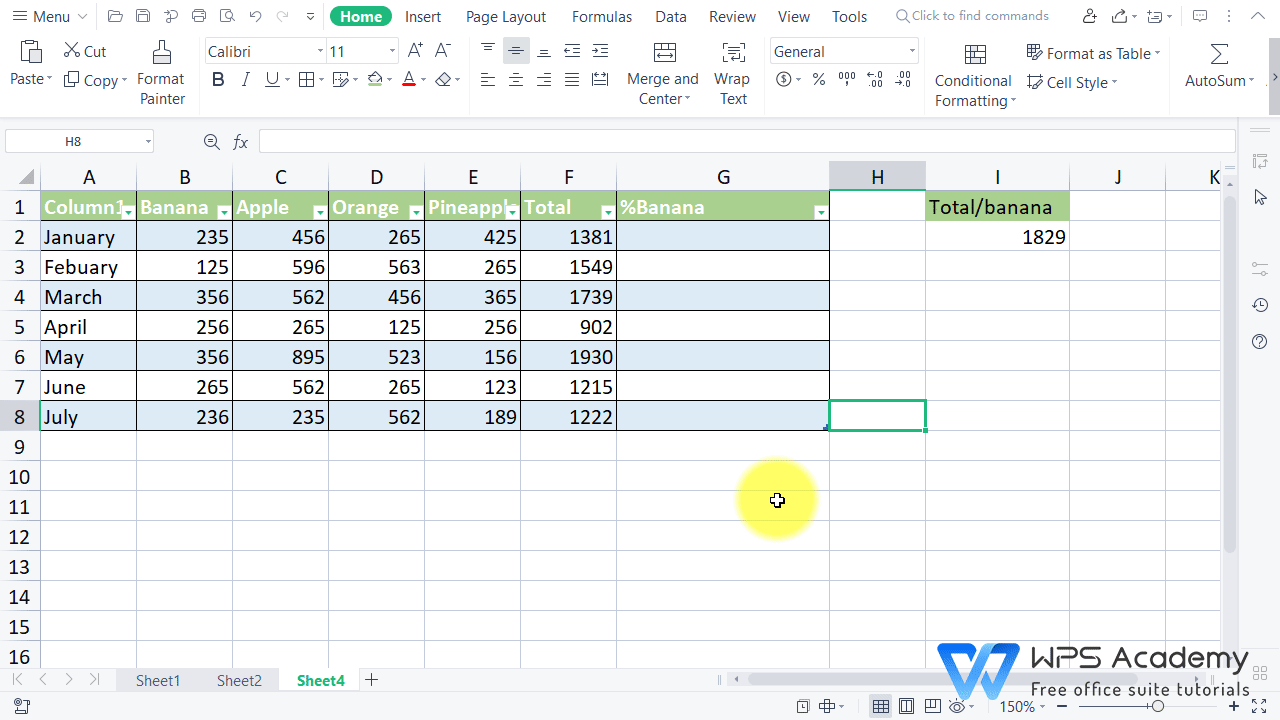With these steps, you have mastered how to use structured references efficiently.